# How to get the current Date and Time in Python

Python is a popular programming language widely used in today’s world providing versatility in different related sectors. One of the cool things that we can do with python is to get the current date and time.

Using the datetime module which is preinstalled in python, we can manipulate date and time like getting the current date and time in different ways. In this article, we are going to show the different examples to get the current date and time in python.

## Get the date and time using datetime module and strftime method()

One of the ways to print the date and time is to use the datetime module and strftime() method. Check the example below for further details.

`\$ sudo vim sample.py`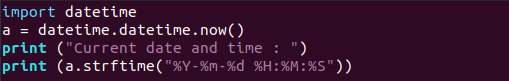```import datetime
a = datetime.datetime.now()
print ("Current date and time : ")
print (a.strftime("%Y-%m-%d %H:%M:%S"))```

Save and close it.

Output:

`\$ python sample1.py`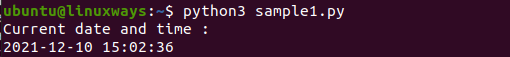Here, you can see the date and time as an output with the use of the datetime module and strftime() method.

## How to get date and time using datetime module

You can use the datetime module and print out the date and time. Check the example below for further details.

`\$ sudo vim sample2.py`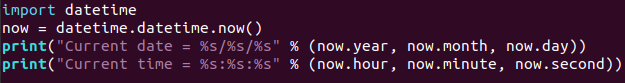```import datetime
now = datetime.datetime.now()
print("Current date = %s/%s/%s" % (now.year, now.month, now.day))
print("Current time = %s:%s:%s" % (now.hour, now.minute, now.second))```

Output:

`\$ python sample2.py`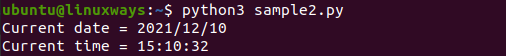Here you can interchange between the year, month and day so it can be displayed as per your wish. For example if you want to display day first and year at last then change the line as shown below.

`print("Current date = %s/%s/%s" % (now.day, now.month, now.year))`

## Get current date and time in different formats using date class

You can display the current date and time in various formats by simply tweaking the code. You can check the below example for further details.

`\$ sudo vim sample3.py`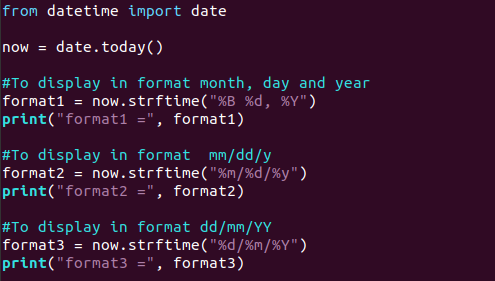```from datetime import date

now = date.today()

#To display in format month, day and year
format1 = now.strftime("%B %d, %Y")
print("format1 =", format1)

#To display in format mm/dd/y
format2 = now.strftime("%m/%d/%y")
print("format2 =", format2)

#To display in format dd/mm/YY
format3 = now.strftime("%d/%m/%Y")
print("format3 =", format3)```

Output:

`\$ python sample3.py`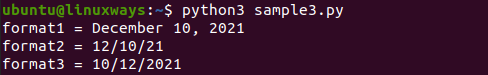## Get current date and time of particular timezone using pytz library

You can get the date and time of a particular time zone and area using the datetime and pytz library. To be clear, check the example below .

`\$ sudo vim sample4.py`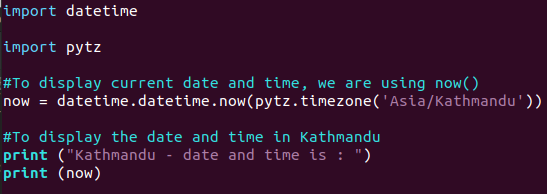```import datetime

import pytz

#To display current date and time, we are using now()
now = datetime.datetime.now(pytz.timezone('Asia/Kathmandu'))

#To display the date and time in Kathmandu
print ("Kathmandu - date and time is : ")

print (now)```

Output:

`\$ python3 sample4.py`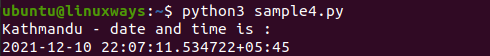You can see the date and time with the details on timezone by using the pytz library. You can simply install pytz with the following command if it is already not installed.

`\$ pip install pytz`

## Get the current date using class date

You can simply check the current date by using the class date from the datetime module.

For further details, you can check the example below.

`\$ sudo vim sample5.py`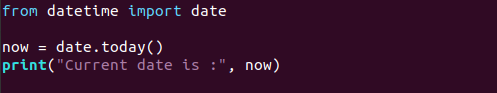```from datetime import date

now = date.today()

print("Current date is :", now)```

Output:

`\$ python3 sample5.py`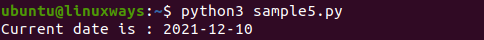Here, you can see the current date with the use of date.today() method. It is one of the simplest and easiest ways to get the current date.

## Conclusion

In this article, you learned to get the current date and time by using some of the python module, class and method. You can also try by using the above examples to check your current date and time. Thank you!